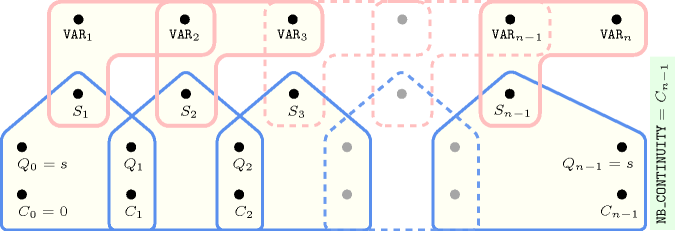## 5.62. change_continuity

Origin

N. Beldiceanu

Constraint

$\mathrm{𝚌𝚑𝚊𝚗𝚐𝚎}_\mathrm{𝚌𝚘𝚗𝚝𝚒𝚗𝚞𝚒𝚝𝚢}\left(\begin{array}{c}\mathrm{𝙽𝙱}_\mathrm{𝙿𝙴𝚁𝙸𝙾𝙳}_\mathrm{𝙲𝙷𝙰𝙽𝙶𝙴},\hfill \\ \mathrm{𝙽𝙱}_\mathrm{𝙿𝙴𝚁𝙸𝙾𝙳}_\mathrm{𝙲𝙾𝙽𝚃𝙸𝙽𝚄𝙸𝚃𝚈},\hfill \\ \mathrm{𝙼𝙸𝙽}_\mathrm{𝚂𝙸𝚉𝙴}_\mathrm{𝙲𝙷𝙰𝙽𝙶𝙴},\hfill \\ \mathrm{𝙼𝙰𝚇}_\mathrm{𝚂𝙸𝚉𝙴}_\mathrm{𝙲𝙷𝙰𝙽𝙶𝙴},\hfill \\ \mathrm{𝙼𝙸𝙽}_\mathrm{𝚂𝙸𝚉𝙴}_\mathrm{𝙲𝙾𝙽𝚃𝙸𝙽𝚄𝙸𝚃𝚈},\hfill \\ \mathrm{𝙼𝙰𝚇}_\mathrm{𝚂𝙸𝚉𝙴}_\mathrm{𝙲𝙾𝙽𝚃𝙸𝙽𝚄𝙸𝚃𝚈},\hfill \\ \mathrm{𝙽𝙱}_\mathrm{𝙲𝙷𝙰𝙽𝙶𝙴},\hfill \\ \mathrm{𝙽𝙱}_\mathrm{𝙲𝙾𝙽𝚃𝙸𝙽𝚄𝙸𝚃𝚈},\hfill \\ \mathrm{𝚅𝙰𝚁𝙸𝙰𝙱𝙻𝙴𝚂},\hfill \\ \mathrm{𝙲𝚃𝚁}\hfill \end{array}\right)$

Arguments
 $\mathrm{𝙽𝙱}_\mathrm{𝙿𝙴𝚁𝙸𝙾𝙳}_\mathrm{𝙲𝙷𝙰𝙽𝙶𝙴}$ $\mathrm{𝚍𝚟𝚊𝚛}$ $\mathrm{𝙽𝙱}_\mathrm{𝙿𝙴𝚁𝙸𝙾𝙳}_\mathrm{𝙲𝙾𝙽𝚃𝙸𝙽𝚄𝙸𝚃𝚈}$ $\mathrm{𝚍𝚟𝚊𝚛}$ $\mathrm{𝙼𝙸𝙽}_\mathrm{𝚂𝙸𝚉𝙴}_\mathrm{𝙲𝙷𝙰𝙽𝙶𝙴}$ $\mathrm{𝚍𝚟𝚊𝚛}$ $\mathrm{𝙼𝙰𝚇}_\mathrm{𝚂𝙸𝚉𝙴}_\mathrm{𝙲𝙷𝙰𝙽𝙶𝙴}$ $\mathrm{𝚍𝚟𝚊𝚛}$ $\mathrm{𝙼𝙸𝙽}_\mathrm{𝚂𝙸𝚉𝙴}_\mathrm{𝙲𝙾𝙽𝚃𝙸𝙽𝚄𝙸𝚃𝚈}$ $\mathrm{𝚍𝚟𝚊𝚛}$ $\mathrm{𝙼𝙰𝚇}_\mathrm{𝚂𝙸𝚉𝙴}_\mathrm{𝙲𝙾𝙽𝚃𝙸𝙽𝚄𝙸𝚃𝚈}$ $\mathrm{𝚍𝚟𝚊𝚛}$ $\mathrm{𝙽𝙱}_\mathrm{𝙲𝙷𝙰𝙽𝙶𝙴}$ $\mathrm{𝚍𝚟𝚊𝚛}$ $\mathrm{𝙽𝙱}_\mathrm{𝙲𝙾𝙽𝚃𝙸𝙽𝚄𝙸𝚃𝚈}$ $\mathrm{𝚍𝚟𝚊𝚛}$ $\mathrm{𝚅𝙰𝚁𝙸𝙰𝙱𝙻𝙴𝚂}$ $\mathrm{𝚌𝚘𝚕𝚕𝚎𝚌𝚝𝚒𝚘𝚗}\left(\mathrm{𝚟𝚊𝚛}-\mathrm{𝚍𝚟𝚊𝚛}\right)$ $\mathrm{𝙲𝚃𝚁}$ $\mathrm{𝚊𝚝𝚘𝚖}$
Restrictions
 $\mathrm{𝙽𝙱}_\mathrm{𝙿𝙴𝚁𝙸𝙾𝙳}_\mathrm{𝙲𝙷𝙰𝙽𝙶𝙴}\ge 0$ $\mathrm{𝙽𝙱}_\mathrm{𝙿𝙴𝚁𝙸𝙾𝙳}_\mathrm{𝙲𝙾𝙽𝚃𝙸𝙽𝚄𝙸𝚃𝚈}\ge 0$ $\mathrm{𝙼𝙸𝙽}_\mathrm{𝚂𝙸𝚉𝙴}_\mathrm{𝙲𝙷𝙰𝙽𝙶𝙴}\ge 0$ $\mathrm{𝙼𝙰𝚇}_\mathrm{𝚂𝙸𝚉𝙴}_\mathrm{𝙲𝙷𝙰𝙽𝙶𝙴}\ge \mathrm{𝙼𝙸𝙽}_\mathrm{𝚂𝙸𝚉𝙴}_\mathrm{𝙲𝙷𝙰𝙽𝙶𝙴}$ $\mathrm{𝙼𝙸𝙽}_\mathrm{𝚂𝙸𝚉𝙴}_\mathrm{𝙲𝙾𝙽𝚃𝙸𝙽𝚄𝙸𝚃𝚈}\ge 0$ $\mathrm{𝙼𝙰𝚇}_\mathrm{𝚂𝙸𝚉𝙴}_\mathrm{𝙲𝙾𝙽𝚃𝙸𝙽𝚄𝙸𝚃𝚈}\ge \mathrm{𝙼𝙸𝙽}_\mathrm{𝚂𝙸𝚉𝙴}_\mathrm{𝙲𝙾𝙽𝚃𝙸𝙽𝚄𝙸𝚃𝚈}$ $\mathrm{𝙽𝙱}_\mathrm{𝙲𝙷𝙰𝙽𝙶𝙴}\ge 0$ $\mathrm{𝙽𝙱}_\mathrm{𝙲𝙾𝙽𝚃𝙸𝙽𝚄𝙸𝚃𝚈}\ge 0$ $\mathrm{𝚛𝚎𝚚𝚞𝚒𝚛𝚎𝚍}$$\left(\mathrm{𝚅𝙰𝚁𝙸𝙰𝙱𝙻𝙴𝚂},\mathrm{𝚟𝚊𝚛}\right)$ $\mathrm{𝙲𝚃𝚁}\in \left[=,\ne ,<,\ge ,>,\le \right]$
Purpose

On the one hand a change is defined by the fact that constraint $\mathrm{𝚅𝙰𝚁𝙸𝙰𝙱𝙻𝙴𝚂}\left[i\right].\mathrm{𝚟𝚊𝚛}\mathrm{𝙲𝚃𝚁}\mathrm{𝚅𝙰𝚁𝙸𝙰𝙱𝙻𝙴𝚂}\left[i+1\right].\mathrm{𝚟𝚊𝚛}$ holds.

On the other hand a continuity is defined by the fact that constraint $\mathrm{𝚅𝙰𝚁𝙸𝙰𝙱𝙻𝙴𝚂}\left[i\right].\mathrm{𝚟𝚊𝚛}\mathrm{𝙲𝚃𝚁}\mathrm{𝚅𝙰𝚁𝙸𝙰𝙱𝙻𝙴𝚂}\left[i+1\right].\mathrm{𝚟𝚊𝚛}$ does not hold.

A period of change on variables

$\mathrm{𝚅𝙰𝚁𝙸𝙰𝙱𝙻𝙴𝚂}\left[i\right].\mathrm{𝚟𝚊𝚛},\mathrm{𝚅𝙰𝚁𝙸𝙰𝙱𝙻𝙴𝚂}\left[i+1\right].\mathrm{𝚟𝚊𝚛},\cdots ,\mathrm{𝚅𝙰𝚁𝙸𝙰𝙱𝙻𝙴𝚂}\left[j\right].\mathrm{𝚟𝚊𝚛}\left(i

is defined by the fact that all constraints $\mathrm{𝚅𝙰𝚁𝙸𝙰𝙱𝙻𝙴𝚂}\left[k\right].\mathrm{𝚟𝚊𝚛}\mathrm{𝙲𝚃𝚁}\mathrm{𝚅𝙰𝚁𝙸𝙰𝙱𝙻𝙴𝚂}\left[k+1\right].\mathrm{𝚟𝚊𝚛}$ hold for $k\in \left[i,j-1\right]$.

A period of continuity on variables

$\mathrm{𝚅𝙰𝚁𝙸𝙰𝙱𝙻𝙴𝚂}\left[i\right].\mathrm{𝚟𝚊𝚛},\mathrm{𝚅𝙰𝚁𝙸𝙰𝙱𝙻𝙴𝚂}\left[i+1\right].\mathrm{𝚟𝚊𝚛},\cdots ,\mathrm{𝚅𝙰𝚁𝙸𝙰𝙱𝙻𝙴𝚂}\left[j\right].\mathrm{𝚟𝚊𝚛}\left(i

is defined by the fact that all constraints $\mathrm{𝚅𝙰𝚁𝙸𝙰𝙱𝙻𝙴𝚂}\left[k\right].\mathrm{𝚟𝚊𝚛}\mathrm{𝙲𝚃𝚁}\mathrm{𝚅𝙰𝚁𝙸𝙰𝙱𝙻𝙴𝚂}\left[k+1\right].\mathrm{𝚟𝚊𝚛}$ do not hold for $k\in \left[i,j-1\right]$.

The constraint $\mathrm{𝚌𝚑𝚊𝚗𝚐𝚎}_\mathrm{𝚌𝚘𝚗𝚝𝚒𝚗𝚞𝚒𝚝𝚢}$ holds if and only if:

• $\mathrm{𝙽𝙱}_\mathrm{𝙿𝙴𝚁𝙸𝙾𝙳}_\mathrm{𝙲𝙷𝙰𝙽𝙶𝙴}$ is equal to the number of periods of change,

• $\mathrm{𝙽𝙱}_\mathrm{𝙿𝙴𝚁𝙸𝙾𝙳}_\mathrm{𝙲𝙾𝙽𝚃𝙸𝙽𝚄𝙸𝚃𝚈}$ is equal to the number of periods of continuity,

• $\mathrm{𝙼𝙸𝙽}_\mathrm{𝚂𝙸𝚉𝙴}_\mathrm{𝙲𝙷𝙰𝙽𝙶𝙴}$ is equal to the number of variables of the smallest period of change,

• $\mathrm{𝙼𝙰𝚇}_\mathrm{𝚂𝙸𝚉𝙴}_\mathrm{𝙲𝙷𝙰𝙽𝙶𝙴}$ is equal to the number of variables of the largest period of change,

• $\mathrm{𝙼𝙸𝙽}_\mathrm{𝚂𝙸𝚉𝙴}_\mathrm{𝙲𝙾𝙽𝚃𝙸𝙽𝚄𝙸𝚃𝚈}$ is equal to the number of variables of the smallest period of continuity,

• $\mathrm{𝙼𝙰𝚇}_\mathrm{𝚂𝙸𝚉𝙴}_\mathrm{𝙲𝙾𝙽𝚃𝙸𝙽𝚄𝙸𝚃𝚈}$ is equal to the number of variables of the largest period of continuity,

• $\mathrm{𝙽𝙱}_\mathrm{𝙲𝙷𝙰𝙽𝙶𝙴}$ is equal to the total number of changes,

• $\mathrm{𝙽𝙱}_\mathrm{𝙲𝙾𝙽𝚃𝙸𝙽𝚄𝙸𝚃𝚈}$ is equal to the total number of continuities.

Example
$\left(3,2,2,4,2,4,6,4,〈1,3,1,8,8,4,7,7,7,7,2〉,\ne \right)$

Figure 5.62.1 makes clear the different parameters that are associated with the given example for the collection $\mathrm{𝚅𝙰𝚁𝙸𝙰𝙱𝙻𝙴𝚂}=〈1,3,1,8,8,4,7,7,7,7,2〉$. We place character | for representing a change and a blank for a continuity. On top of the solution we represent the different periods of change, while below we show the different periods of continuity. The $\mathrm{𝚌𝚑𝚊𝚗𝚐𝚎}_\mathrm{𝚌𝚘𝚗𝚝𝚒𝚗𝚞𝚒𝚝𝚢}$ constraint holds since:

• Its number of periods of change $\mathrm{𝙽𝙱}_\mathrm{𝙿𝙴𝚁𝙸𝙾𝙳}_\mathrm{𝙲𝙷𝙰𝙽𝙶𝙴}$ is equal to 3 (i.e., the 3 periods depicted on top of Figure 5.62.1),

• Its number of periods of continuity $\mathrm{𝙽𝙱}_\mathrm{𝙿𝙴𝚁𝙸𝙾𝙳}_\mathrm{𝙲𝙾𝙽𝚃𝙸𝙽𝚄𝙸𝚃𝚈}$ is equal to 2 (i.e., the 2 periods depicted below Figure 5.62.1),

• The number of variables of its smallest period of change $\mathrm{𝙼𝙸𝙽}_\mathrm{𝚂𝙸𝚉𝙴}_\mathrm{𝙲𝙷𝙰𝙽𝙶𝙴}$ is equal to 2 (i.e., the number of variables involved in the third period of change $72$ depicted on top of Figure 5.62.1),

• The number of variables of the largest period of change $\mathrm{𝙼𝙰𝚇}_\mathrm{𝚂𝙸𝚉𝙴}_\mathrm{𝙲𝙷𝙰𝙽𝙶𝙴}$ is equal to 4 (i.e., the number of variables involved in the first period of change $1318$ depicted on top of Figure 5.62.1),

• The number of variables of the smallest period of continuity $\mathrm{𝙼𝙸𝙽}_\mathrm{𝚂𝙸𝚉𝙴}_\mathrm{𝙲𝙾𝙽𝚃𝙸𝙽𝚄𝙸𝚃𝚈}$ is equal to 2 (i.e., the number of variables involved in the first period $88$ depicted below Figure 5.62.1),

• The number of variables of the largest period of continuity $\mathrm{𝙼𝙰𝚇}_\mathrm{𝚂𝙸𝚉𝙴}_\mathrm{𝙲𝙾𝙽𝚃𝙸𝙽𝚄𝙸𝚃𝚈}$ is equal to 4 (i.e., the number of variables involved in the second period $7777$ depicted below Figure 5.62.1),

• The total number of changes $\mathrm{𝙽𝙱}_\mathrm{𝙲𝙷𝙰𝙽𝙶𝙴}$ is equal to 6 (i.e., the number of occurrences of character | in Figure 5.62.1),

• The total number of continuities $\mathrm{𝙽𝙱}_\mathrm{𝙲𝙾𝙽𝚃𝙸𝙽𝚄𝙸𝚃𝚈}$ is equal to 4.

##### Figure 5.62.1. Illustration of the Example slot: periods of changes and periods of continuities wrt the constraint $\mathrm{𝙲𝚃𝚁}$ equal to $\ne$Typical
 $\mathrm{𝙽𝙱}_\mathrm{𝙿𝙴𝚁𝙸𝙾𝙳}_\mathrm{𝙲𝙷𝙰𝙽𝙶𝙴}>0$ $\mathrm{𝙽𝙱}_\mathrm{𝙿𝙴𝚁𝙸𝙾𝙳}_\mathrm{𝙲𝙾𝙽𝚃𝙸𝙽𝚄𝙸𝚃𝚈}>0$ $\mathrm{𝙼𝙸𝙽}_\mathrm{𝚂𝙸𝚉𝙴}_\mathrm{𝙲𝙷𝙰𝙽𝙶𝙴}>0$ $\mathrm{𝙼𝙸𝙽}_\mathrm{𝚂𝙸𝚉𝙴}_\mathrm{𝙲𝙾𝙽𝚃𝙸𝙽𝚄𝙸𝚃𝚈}>0$ $\mathrm{𝙽𝙱}_\mathrm{𝙲𝙷𝙰𝙽𝙶𝙴}>0$ $\mathrm{𝙽𝙱}_\mathrm{𝙲𝙾𝙽𝚃𝙸𝙽𝚄𝙸𝚃𝚈}>0$ $|\mathrm{𝚅𝙰𝚁𝙸𝙰𝙱𝙻𝙴𝚂}|>1$ $\mathrm{𝚛𝚊𝚗𝚐𝚎}$$\left(\mathrm{𝚅𝙰𝚁𝙸𝙰𝙱𝙻𝙴𝚂}.\mathrm{𝚟𝚊𝚛}\right)>1$ $\mathrm{𝙲𝚃𝚁}\in \left[\ne \right]$
Symmetry

One and the same constant can be added to the $\mathrm{𝚟𝚊𝚛}$ attribute of all items of $\mathrm{𝚅𝙰𝚁𝙸𝙰𝙱𝙻𝙴𝚂}$.

Arg. properties
• Functional dependency: $\mathrm{𝙽𝙱}_\mathrm{𝙿𝙴𝚁𝙸𝙾𝙳}_\mathrm{𝙲𝙷𝙰𝙽𝙶𝙴}$ determined by $\mathrm{𝚅𝙰𝚁𝙸𝙰𝙱𝙻𝙴𝚂}$ and $\mathrm{𝙲𝚃𝚁}$.

• Functional dependency: $\mathrm{𝙽𝙱}_\mathrm{𝙿𝙴𝚁𝙸𝙾𝙳}_\mathrm{𝙲𝙾𝙽𝚃𝙸𝙽𝚄𝙸𝚃𝚈}$ determined by $\mathrm{𝚅𝙰𝚁𝙸𝙰𝙱𝙻𝙴𝚂}$ and $\mathrm{𝙲𝚃𝚁}$.

• Functional dependency: $\mathrm{𝙼𝙸𝙽}_\mathrm{𝚂𝙸𝚉𝙴}_\mathrm{𝙲𝙷𝙰𝙽𝙶𝙴}$ determined by $\mathrm{𝚅𝙰𝚁𝙸𝙰𝙱𝙻𝙴𝚂}$ and $\mathrm{𝙲𝚃𝚁}$.

• Functional dependency: $\mathrm{𝙼𝙰𝚇}_\mathrm{𝚂𝙸𝚉𝙴}_\mathrm{𝙲𝙷𝙰𝙽𝙶𝙴}$ determined by $\mathrm{𝚅𝙰𝚁𝙸𝙰𝙱𝙻𝙴𝚂}$ and $\mathrm{𝙲𝚃𝚁}$.

• Functional dependency: $\mathrm{𝙼𝙸𝙽}_\mathrm{𝚂𝙸𝚉𝙴}_\mathrm{𝙲𝙾𝙽𝚃𝙸𝙽𝚄𝙸𝚃𝚈}$ determined by $\mathrm{𝚅𝙰𝚁𝙸𝙰𝙱𝙻𝙴𝚂}$ and $\mathrm{𝙲𝚃𝚁}$.

• Functional dependency: $\mathrm{𝙼𝙰𝚇}_\mathrm{𝚂𝙸𝚉𝙴}_\mathrm{𝙲𝙾𝙽𝚃𝙸𝙽𝚄𝙸𝚃𝚈}$ determined by $\mathrm{𝚅𝙰𝚁𝙸𝙰𝙱𝙻𝙴𝚂}$ and $\mathrm{𝙲𝚃𝚁}$.

• Functional dependency: $\mathrm{𝙽𝙱}_\mathrm{𝙲𝙷𝙰𝙽𝙶𝙴}$ determined by $\mathrm{𝚅𝙰𝚁𝙸𝙰𝙱𝙻𝙴𝚂}$ and $\mathrm{𝙲𝚃𝚁}$.

• Functional dependency: $\mathrm{𝙽𝙱}_\mathrm{𝙲𝙾𝙽𝚃𝙸𝙽𝚄𝙸𝚃𝚈}$ determined by $\mathrm{𝚅𝙰𝚁𝙸𝙰𝙱𝙻𝙴𝚂}$ and $\mathrm{𝙲𝚃𝚁}$.

Remark

If the variables of the collection $\mathrm{𝚅𝙰𝚁𝙸𝙰𝙱𝙻𝙴𝚂}$ have to take distinct values between 1 and the total number of variables, we have what is called a permutation. In this case, if we choose the binary constraint $<$, then $\mathrm{𝙼𝙰𝚇}_\mathrm{𝚂𝙸𝚉𝙴}_\mathrm{𝙲𝙷𝙰𝙽𝙶𝙴}$ gives the size of the longest run of the permutation; A run is a maximal increasing contiguous subsequence in a permutation.

Keywords
Arc input(s)

$\mathrm{𝚅𝙰𝚁𝙸𝙰𝙱𝙻𝙴𝚂}$

Arc generator
$\mathrm{𝑃𝐴𝑇𝐻}$$↦\mathrm{𝚌𝚘𝚕𝚕𝚎𝚌𝚝𝚒𝚘𝚗}\left(\mathrm{𝚟𝚊𝚛𝚒𝚊𝚋𝚕𝚎𝚜}\mathtt{1},\mathrm{𝚟𝚊𝚛𝚒𝚊𝚋𝚕𝚎𝚜}\mathtt{2}\right)$

Arc arity
Arc constraint(s)
$\mathrm{𝚟𝚊𝚛𝚒𝚊𝚋𝚕𝚎𝚜}\mathtt{1}.\mathrm{𝚟𝚊𝚛}\mathrm{𝙲𝚃𝚁}\mathrm{𝚟𝚊𝚛𝚒𝚊𝚋𝚕𝚎𝚜}\mathtt{2}.\mathrm{𝚟𝚊𝚛}$
Graph property(ies)
 $•$$\mathrm{𝐍𝐂𝐂}$$=\mathrm{𝙽𝙱}_\mathrm{𝙿𝙴𝚁𝙸𝙾𝙳}_\mathrm{𝙲𝙷𝙰𝙽𝙶𝙴}$ $•$$\mathrm{𝐌𝐈𝐍}_\mathrm{𝐍𝐂𝐂}$$=\mathrm{𝙼𝙸𝙽}_\mathrm{𝚂𝙸𝚉𝙴}_\mathrm{𝙲𝙷𝙰𝙽𝙶𝙴}$ $•$$\mathrm{𝐌𝐀𝐗}_\mathrm{𝐍𝐂𝐂}$$=\mathrm{𝙼𝙰𝚇}_\mathrm{𝚂𝙸𝚉𝙴}_\mathrm{𝙲𝙷𝙰𝙽𝙶𝙴}$ $•$$\mathrm{𝐍𝐀𝐑𝐂}$$=\mathrm{𝙽𝙱}_\mathrm{𝙲𝙷𝙰𝙽𝙶𝙴}$

Graph class
 $•$$\mathrm{𝙰𝙲𝚈𝙲𝙻𝙸𝙲}$ $•$$\mathrm{𝙱𝙸𝙿𝙰𝚁𝚃𝙸𝚃𝙴}$ $•$$\mathrm{𝙽𝙾}_\mathrm{𝙻𝙾𝙾𝙿}$

Arc input(s)

$\mathrm{𝚅𝙰𝚁𝙸𝙰𝙱𝙻𝙴𝚂}$

Arc generator
$\mathrm{𝑃𝐴𝑇𝐻}$$↦\mathrm{𝚌𝚘𝚕𝚕𝚎𝚌𝚝𝚒𝚘𝚗}\left(\mathrm{𝚟𝚊𝚛𝚒𝚊𝚋𝚕𝚎𝚜}\mathtt{1},\mathrm{𝚟𝚊𝚛𝚒𝚊𝚋𝚕𝚎𝚜}\mathtt{2}\right)$

Arc arity
Arc constraint(s)
$\mathrm{𝚟𝚊𝚛𝚒𝚊𝚋𝚕𝚎𝚜}\mathtt{1}.\mathrm{𝚟𝚊𝚛}¬\mathrm{𝙲𝚃𝚁}\mathrm{𝚟𝚊𝚛𝚒𝚊𝚋𝚕𝚎𝚜}\mathtt{2}.\mathrm{𝚟𝚊𝚛}$
Graph property(ies)
 $•$$\mathrm{𝐍𝐂𝐂}$$=\mathrm{𝙽𝙱}_\mathrm{𝙿𝙴𝚁𝙸𝙾𝙳}_\mathrm{𝙲𝙾𝙽𝚃𝙸𝙽𝚄𝙸𝚃𝚈}$ $•$$\mathrm{𝐌𝐈𝐍}_\mathrm{𝐍𝐂𝐂}$$=\mathrm{𝙼𝙸𝙽}_\mathrm{𝚂𝙸𝚉𝙴}_\mathrm{𝙲𝙾𝙽𝚃𝙸𝙽𝚄𝙸𝚃𝚈}$ $•$$\mathrm{𝐌𝐀𝐗}_\mathrm{𝐍𝐂𝐂}$$=\mathrm{𝙼𝙰𝚇}_\mathrm{𝚂𝙸𝚉𝙴}_\mathrm{𝙲𝙾𝙽𝚃𝙸𝙽𝚄𝙸𝚃𝚈}$ $•$$\mathrm{𝐍𝐀𝐑𝐂}$$=\mathrm{𝙽𝙱}_\mathrm{𝙲𝙾𝙽𝚃𝙸𝙽𝚄𝙸𝚃𝚈}$

Graph class
 $•$$\mathrm{𝙰𝙲𝚈𝙲𝙻𝙸𝙲}$ $•$$\mathrm{𝙱𝙸𝙿𝙰𝚁𝚃𝙸𝚃𝙴}$ $•$$\mathrm{𝙽𝙾}_\mathrm{𝙻𝙾𝙾𝙿}$

Graph model

We use two graph constraints to respectively catch the constraints on the period of changes and of the period of continuities. In both case each period corresponds to a connected component of the final graph.

Parts (A) and (B) of Figure 5.62.2 respectively show the initial and final graph associated with the first graph constraint of the Example slot.

##### Figure 5.62.2. Initial and final graph of the $\mathrm{𝚌𝚑𝚊𝚗𝚐𝚎}_\mathrm{𝚌𝚘𝚗𝚝𝚒𝚗𝚞𝚒𝚝𝚢}$ constraint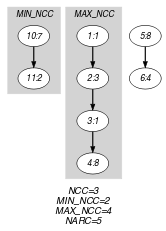(a) (b)
Automaton

Figures 5.62.35.62.45.62.75.62.85.62.115.62.12 and 5.62.15 depict the automata associated with the different graph parameters of the $\mathrm{𝚌𝚑𝚊𝚗𝚐𝚎}_\mathrm{𝚌𝚘𝚗𝚝𝚒𝚗𝚞𝚒𝚝𝚢}$ constraint. For the automata that respectively compute $\mathrm{𝙽𝙱}_\mathrm{𝙿𝙴𝚁𝙸𝙾𝙳}_\mathrm{𝙲𝙷𝙰𝙽𝙶𝙴}$, $\mathrm{𝙽𝙱}_\mathrm{𝙿𝙴𝚁𝙸𝙾𝙳}_\mathrm{𝙲𝙾𝙽𝚃𝙸𝙽𝚄𝙸𝚃𝚈}$ $\mathrm{𝙼𝙸𝙽}_\mathrm{𝚂𝙸𝚉𝙴}_\mathrm{𝙲𝙷𝙰𝙽𝙶𝙴}$, $\mathrm{𝙼𝙸𝙽}_\mathrm{𝚂𝙸𝚉𝙴}_\mathrm{𝙲𝙾𝙽𝚃𝙸𝙽𝚄𝙸𝚃𝚈}$ $\mathrm{𝙼𝙰𝚇}_\mathrm{𝚂𝙸𝚉𝙴}_\mathrm{𝙲𝙷𝙰𝙽𝙶𝙴}$, $\mathrm{𝙼𝙰𝚇}_\mathrm{𝚂𝙸𝚉𝙴}_\mathrm{𝙲𝙾𝙽𝚃𝙸𝙽𝚄𝙸𝚃𝚈}$ $\mathrm{𝙽𝙱}_\mathrm{𝙲𝙷𝙰𝙽𝙶𝙴}$ and $\mathrm{𝙽𝙱}_\mathrm{𝙲𝙾𝙽𝚃𝙸𝙽𝚄𝙸𝚃𝚈}$ we have a 0-1 signature variable ${S}_{i}$ for each pair of consecutive variables $\left({\mathrm{𝚅𝙰𝚁}}_{i},{\mathrm{𝚅𝙰𝚁}}_{i+1}\right)$ of the collection $\mathrm{𝚅𝙰𝚁𝙸𝙰𝙱𝙻𝙴𝚂}$. The following signature constraint links ${\mathrm{𝚅𝙰𝚁}}_{i}$, ${\mathrm{𝚅𝙰𝚁}}_{i+1}$ and ${S}_{i}$: ${\mathrm{𝚅𝙰𝚁}}_{i}\mathrm{𝙲𝚃𝚁}{\mathrm{𝚅𝙰𝚁}}_{i+1}⇔{S}_{i}$.

##### Figure 5.62.3. Automaton for the $\mathrm{𝙽𝙱}_\mathrm{𝙿𝙴𝚁𝙸𝙾𝙳}_\mathrm{𝙲𝙷𝙰𝙽𝙶𝙴}$ argument of the $\mathrm{𝚌𝚑𝚊𝚗𝚐𝚎}_\mathrm{𝚌𝚘𝚗𝚝𝚒𝚗𝚞𝚒𝚝𝚢}$ constraint and its glue matrix; note that the reverse of $\mathrm{𝚌𝚑𝚊𝚗𝚐𝚎}_\mathrm{𝚌𝚘𝚗𝚝𝚒𝚗𝚞𝚒𝚝𝚢}$ with $\mathrm{𝙲𝚃𝚁}\in \left\{=,\ne \right\}$ is the same constraint, while the reverse with $\mathrm{𝙲𝚃𝚁}\in \left\{<\right\}$ (resp. $\mathrm{𝙲𝚃𝚁}\in \left\{\le \right\}$) is $\mathrm{𝙲𝚃𝚁}\in \left\{>\right\}$ (resp. $\mathrm{𝙲𝚃𝚁}\in \left\{\ge \right\}$).##### Figure 5.62.4. Automaton for the $\mathrm{𝙽𝙱}_\mathrm{𝙿𝙴𝚁𝙸𝙾𝙳}_\mathrm{𝙲𝙾𝙽𝚃𝙸𝙽𝚄𝙸𝚃𝚈}$ argument of the $\mathrm{𝚌𝚑𝚊𝚗𝚐𝚎}_\mathrm{𝚌𝚘𝚗𝚝𝚒𝚗𝚞𝚒𝚝𝚢}$ constraint and its glue matrix; note that the reverse of $\mathrm{𝚌𝚑𝚊𝚗𝚐𝚎}_\mathrm{𝚌𝚘𝚗𝚝𝚒𝚗𝚞𝚒𝚝𝚢}$ with $\mathrm{𝙲𝚃𝚁}\in \left\{=,\ne \right\}$ is the same constraint, while the reverse with $\mathrm{𝙲𝚃𝚁}\in \left\{<\right\}$ (resp. $\mathrm{𝙲𝚃𝚁}\in \left\{\le \right\}$) is $\mathrm{𝙲𝚃𝚁}\in \left\{>\right\}$ (resp. $\mathrm{𝙲𝚃𝚁}\in \left\{\ge \right\}$).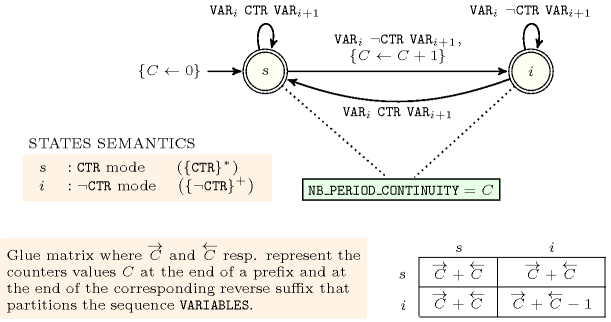##### Figure 5.62.5. Hypergraph of the reformulation corresponding to the automaton of the $\mathrm{𝙽𝙱}_\mathrm{𝙿𝙴𝚁𝙸𝙾𝙳}_\mathrm{𝙲𝙷𝙰𝙽𝙶𝙴}$ argument of the $\mathrm{𝚌𝚑𝚊𝚗𝚐𝚎}_\mathrm{𝚌𝚘𝚗𝚝𝚒𝚗𝚞𝚒𝚝𝚢}$ constraint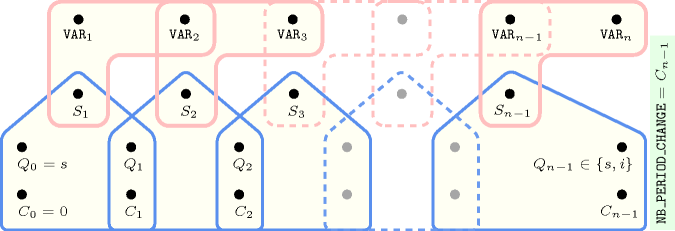##### Figure 5.62.6. Hypergraph of the reformulation corresponding to the automaton of the $\mathrm{𝙽𝙱}_\mathrm{𝙿𝙴𝚁𝙸𝙾𝙳}_\mathrm{𝙲𝙾𝙽𝚃𝙸𝙽𝚄𝙸𝚃𝚈}$ argument of the $\mathrm{𝚌𝚑𝚊𝚗𝚐𝚎}_\mathrm{𝚌𝚘𝚗𝚝𝚒𝚗𝚞𝚒𝚝𝚢}$ constraint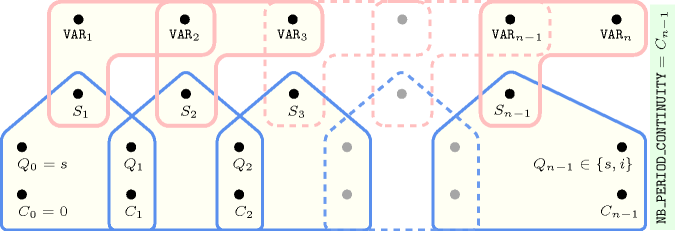##### Figure 5.62.7. Automaton for the $\mathrm{𝙼𝙸𝙽}_\mathrm{𝚂𝙸𝚉𝙴}_\mathrm{𝙲𝙷𝙰𝙽𝙶𝙴}$ argument of the $\mathrm{𝚌𝚑𝚊𝚗𝚐𝚎}_\mathrm{𝚌𝚘𝚗𝚝𝚒𝚗𝚞𝚒𝚝𝚢}$ constraint; its glue matrix when $\mathrm{𝙲𝚃𝚁}\in \left\{=,\ne \right\}$.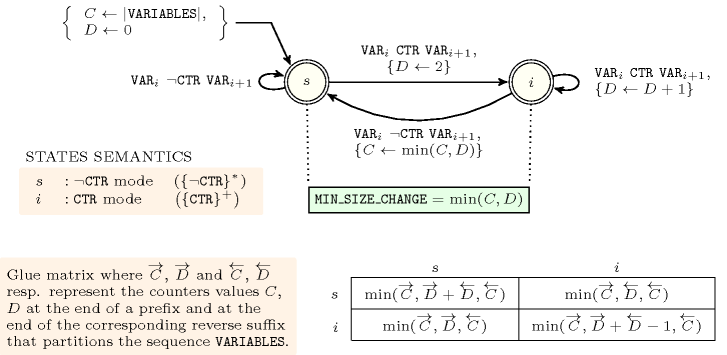##### Figure 5.62.8. Automaton for the $\mathrm{𝙼𝙸𝙽}_\mathrm{𝚂𝙸𝚉𝙴}_\mathrm{𝙲𝙾𝙽𝚃𝙸𝙽𝚄𝙸𝚃𝚈}$ argument of the $\mathrm{𝚌𝚑𝚊𝚗𝚐𝚎}_\mathrm{𝚌𝚘𝚗𝚝𝚒𝚗𝚞𝚒𝚝𝚢}$ constraint; its glue matrix when $\mathrm{𝙲𝚃𝚁}\in \left\{=,\ne \right\}$.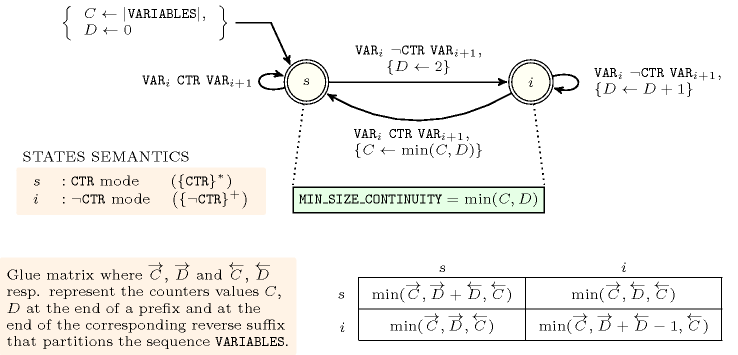##### Figure 5.62.9. Hypergraph of the reformulation corresponding to the automaton of the $\mathrm{𝙼𝙸𝙽}_\mathrm{𝚂𝙸𝚉𝙴}_\mathrm{𝙲𝙷𝙰𝙽𝙶𝙴}$ argument of the $\mathrm{𝚌𝚑𝚊𝚗𝚐𝚎}_\mathrm{𝚌𝚘𝚗𝚝𝚒𝚗𝚞𝚒𝚝𝚢}$ constraint where $𝙽$ stands for $|\mathrm{𝚅𝙰𝚁𝙸𝙰𝙱𝙻𝙴𝚂}|$ (since all states of the automaton are accepting there is no restriction on the last variable ${Q}_{n-1}$)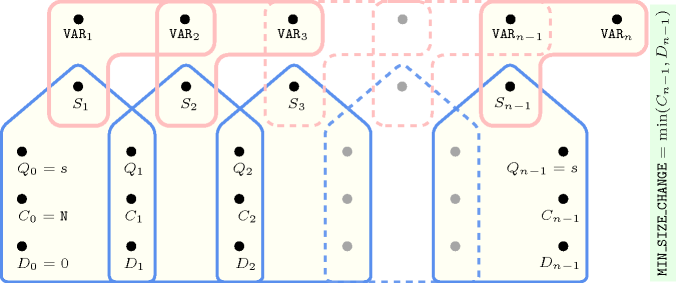##### Figure 5.62.10. Hypergraph of the reformulation corresponding to the automaton of the $\mathrm{𝙼𝙸𝙽}_\mathrm{𝚂𝙸𝚉𝙴}_\mathrm{𝙲𝙾𝙽𝚃𝙸𝙽𝚄𝙸𝚃𝚈}$ argument of the $\mathrm{𝚌𝚑𝚊𝚗𝚐𝚎}_\mathrm{𝚌𝚘𝚗𝚝𝚒𝚗𝚞𝚒𝚝𝚢}$ constraint where $𝙽$ stands for $|\mathrm{𝚅𝙰𝚁𝙸𝙰𝙱𝙻𝙴𝚂}|$ (since all states of the automaton are accepting there is no restriction on the last variable ${Q}_{n-1}$)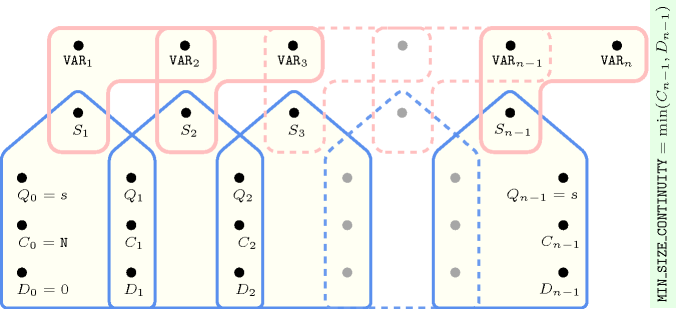##### Figure 5.62.11. Automaton for the $\mathrm{𝙼𝙰𝚇}_\mathrm{𝚂𝙸𝚉𝙴}_\mathrm{𝙲𝙷𝙰𝙽𝙶𝙴}$ argument of the $\mathrm{𝚌𝚑𝚊𝚗𝚐𝚎}_\mathrm{𝚌𝚘𝚗𝚝𝚒𝚗𝚞𝚒𝚝𝚢}$ constraint; its glue matrix when $\mathrm{𝙲𝚃𝚁}\in \left\{=,\ne \right\}$.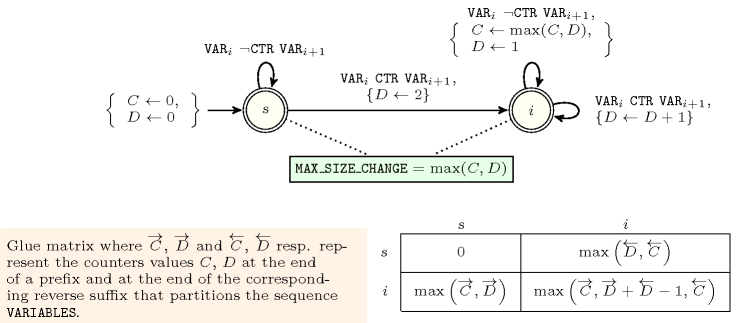##### Figure 5.62.12. Automaton for the $\mathrm{𝙼𝙰𝚇}_\mathrm{𝚂𝙸𝚉𝙴}_\mathrm{𝙲𝙾𝙽𝚃𝙸𝙽𝚄𝙸𝚃𝚈}$ argument of the $\mathrm{𝚌𝚑𝚊𝚗𝚐𝚎}_\mathrm{𝚌𝚘𝚗𝚝𝚒𝚗𝚞𝚒𝚝𝚢}$ constraint; its glue matrix when $\mathrm{𝙲𝚃𝚁}\in \left\{=,\ne \right\}$.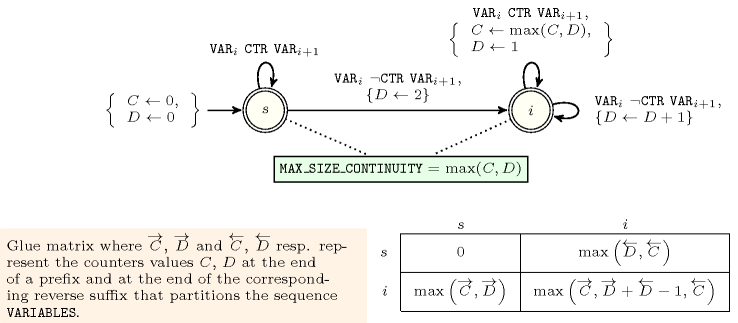##### Figure 5.62.13. Hypergraph of the reformulation corresponding to the automaton of the $\mathrm{𝙼𝙰𝚇}_\mathrm{𝚂𝙸𝚉𝙴}_\mathrm{𝙲𝙷𝙰𝙽𝙶𝙴}$ argument of the $\mathrm{𝚌𝚑𝚊𝚗𝚐𝚎}_\mathrm{𝚌𝚘𝚗𝚝𝚒𝚗𝚞𝚒𝚝𝚢}$ constraint (since all states of the automaton are accepting there is no restriction on the last variable ${Q}_{n-1}$)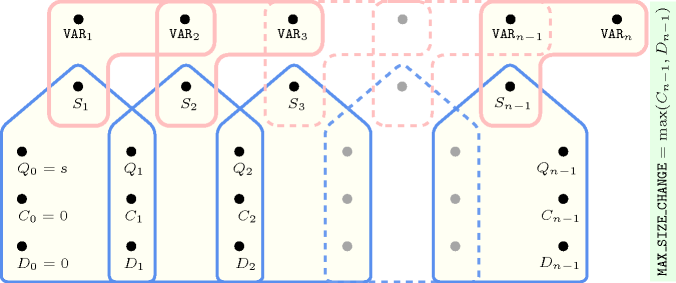##### Figure 5.62.14. Hypergraph of the reformulation corresponding to the automaton of the $\mathrm{𝙼𝙰𝚇}_\mathrm{𝚂𝙸𝚉𝙴}_\mathrm{𝙲𝙾𝙽𝚃𝙸𝙽𝚄𝙸𝚃𝚈}$ argument of the $\mathrm{𝚌𝚑𝚊𝚗𝚐𝚎}_\mathrm{𝚌𝚘𝚗𝚝𝚒𝚗𝚞𝚒𝚝𝚢}$ constraint (since all states of the automaton are accepting there is no restriction on the last variable ${Q}_{n-1}$)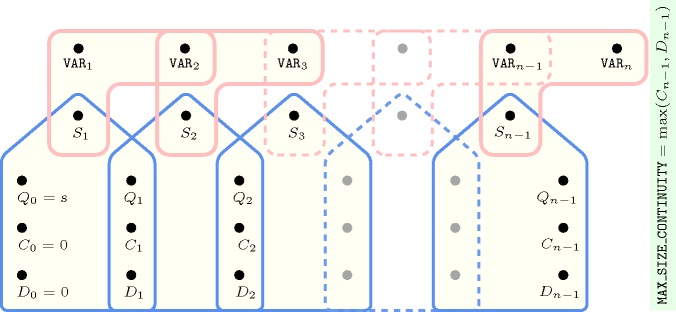##### Figure 5.62.15. Automata for the $\mathrm{𝙽𝙱}_\mathrm{𝙲𝙷𝙰𝙽𝙶𝙴}$ and $\mathrm{𝙽𝙱}_\mathrm{𝙲𝙾𝙽𝚃𝙸𝙽𝚄𝙸𝚃𝚈}$ arguments of the $\mathrm{𝚌𝚑𝚊𝚗𝚐𝚎}_\mathrm{𝚌𝚘𝚗𝚝𝚒𝚗𝚞𝚒𝚝𝚢}$ constraint; their common glue matrix when $argCTR\in \left\{=,\ne \right\}$.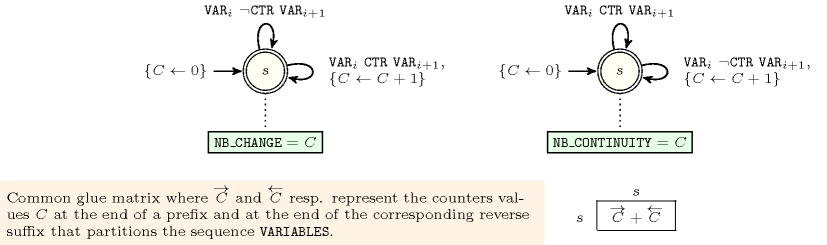##### Figure 5.62.16. Hypergraph of the reformulation corresponding to the automaton of the $\mathrm{𝙽𝙱}_\mathrm{𝙲𝙷𝙰𝙽𝙶𝙴}$ argument of the $\mathrm{𝚌𝚑𝚊𝚗𝚐𝚎}_\mathrm{𝚌𝚘𝚗𝚝𝚒𝚗𝚞𝚒𝚝𝚢}$ constraint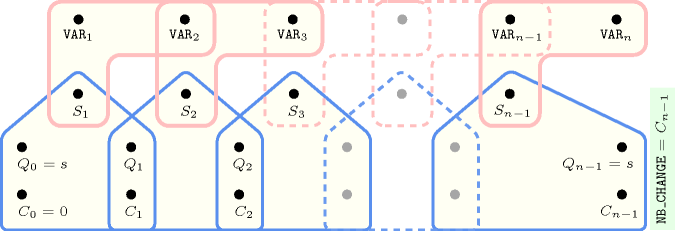##### Figure 5.62.17. Hypergraph of the reformulation corresponding to the automaton of the $\mathrm{𝙽𝙱}_\mathrm{𝙲𝙾𝙽𝚃𝙸𝙽𝚄𝙸𝚃𝚈}$ argument of the $\mathrm{𝚌𝚑𝚊𝚗𝚐𝚎}_\mathrm{𝚌𝚘𝚗𝚝𝚒𝚗𝚞𝚒𝚝𝚢}$ constraint